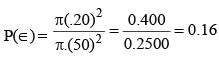Courses

# Test: Arithmetic - 3 (2017-2016)

## 20 Questions MCQ Test UPSC Topic Wise Previous Year Questions | Test: Arithmetic - 3 (2017-2016)

Description
This mock test of Test: Arithmetic - 3 (2017-2016) for UPSC helps you for every UPSC entrance exam. This contains 20 Multiple Choice Questions for UPSC Test: Arithmetic - 3 (2017-2016) (mcq) to study with solutions a complete question bank. The solved questions answers in this Test: Arithmetic - 3 (2017-2016) quiz give you a good mix of easy questions and tough questions. UPSC students definitely take this Test: Arithmetic - 3 (2017-2016) exercise for a better result in the exam. You can find other Test: Arithmetic - 3 (2017-2016) extra questions, long questions & short questions for UPSC on EduRev as well by searching above.
QUESTION: 1

### There are 4 horizontal and 4 vertical lines, parallel and equidistant to one another on a board. What is the maximum number of rectangles and squares that can be formed? 

Solution: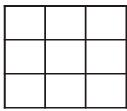Number of squares and rectangles in a 3 × 3 grid = 13 + 23 +33 = 36.

QUESTION: 2

### There is a milk sample with 50% water in it. If 1/3rd of this milk is added to equal amount of pure milk, then water in the new mixture will fall down to 

Solution:

Let the original amount be 150 ml.
According to question.
50 ml of mixture + 50ml of pure milk
⇒ 25 ml of  Milk + 25ml of water + 50 ml of pure milk.
Hence, % of water in new mixture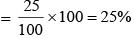.

QUESTION: 3

### There are thirteen 2-digit consecutive odd numbers. If 39 is the mean of the first five such numbers, then what is the mean of all the thirteen numbers? 

Solution:

Since 39 is the mean of first five numbers, first five numbers are
35, 37, 39, 41 and 43 and next 8 numbers are 45, 47, 49, 51, 53, 55, 57 and 59.
Hence, mean = (13 + 1)/2 , 7th number or middle number = 47.

QUESTION: 4

A clock strikes once at 1 o'clock, twice at 2 o'clock and thrice at 3 o'clock, and so on. If it takes 12 seconds to strike at 5 o'clock, what is the time taken by it to strike at 10 o'clock?



Solution:

At 5 O’clock the clock will strike 5 times. It’s given that it takes 12 seconds to do so.
Now, at 10’O clock the clock will strike 10 times.
Hence, the time taken by it to strike = 9 × 3 = 27 seconds.

QUESTION: 5

In a city, 12% of households earn less than ₹ 30,000 per year, 6% households earn more than ₹ 2,00,000 per year, 22% households earn more than ₹ 1,00,000 per year and 990 households earn between ₹ 30,000 and ₹ 1,00,000 per year. How many households earn between ₹ 1,00,000 and ₹ 2,00,000 per year?



Solution:

12% earn less than ₹ 30,000.
6% earn more than ₹ 2,00,000.
22% earn more than ₹ 1,00,000.
So, between ₹ 30,000 – ₹ 1, 00, 000 there are (100 – 22 – 12)% = 66%  household.
66% household is equal to 990
Hence, Number of household = 1500.
Number of household between 1,00,000 and 2,00,000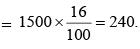QUESTION: 6

A watch loses 2 minutes in every 24 hours. while another watch gains 2 minutes in every 24 hours. At a particular instant, the two watches showed an identical time. Which of the following statements is correct if 24-hour clock is followed?



Solution:

None of the above statement is correct.

QUESTION: 7

Suppose the average weight of 9 persons is 50 kg. The average weight of the first 5 persons is 45 kg, whereas the average weight of the last 5 persons is 55 kg, Then the weight of the 5th person will be



Solution:

The weight of the 5th person = sum of first 5 person + Sum of last five person– Sum of weight of 9 persons.
= 5 × 45 + 5 × 55 – 9 × 50 kg = 50 kg

QUESTION: 8

Gopal bought a cell phone and sold it to Ram at 10% profit. Then Ram wanted to sell it back to Gopal at 10% loss. What will be Gopal's position if he agreed?



Solution:

Let the original price of phone = ₹100
SP of Gopal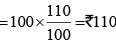SP of Ram = 110 – 10% of 110 = ₹ 99
Total Profit of gopal = ₹100 – ₹ 99 = 1
But profit was made as CP hence, 1% profit.

QUESTION: 9

There are three pillars, X, Y and Z of different heights. Three spiders A, B and C start to climb on these pillars simultaneously. In one chance. A climbs on X by 6cm but slips down 1 cm. B climbs on Y by 7 cm but slips down 3 cm. C climbs on Z by 6.5 cm but slips down 2 cm. If each of the requires 40 chances to reach the top of the pillars, what is the height of the shortest pillar?



Solution:

Distance travelled by A in one chance = (6 –1) cm = 5 cm
Distance travelled by B in one chance = (7 – 3) cm = 4 cm
Distance travelled by C in one chance = (6.5 – 2) cm = 4.5 cm.
Hence, Length of pillar A = 5 × 39 + 6 = 201 cm
Length of pillar B = 4 × 39 +7 = 163 cm
Length of pillar C = 4.5 × 39 + 6.5 = 182 cm
Length of shortest pillar → B. 163 cm.

QUESTION: 10

What is the total number of digits printed, if a book containing 150 pages is to be numebred from 1 to 150



Solution:

Number of digits used For 1 – 9 = 9 × 1 = 9
Number of digits used For 10 – 99 = 90 × 2 = 180
Number of digits used for 100 – 150 = 51 × 3 = 153
Hence, 153 + 180 + 9 = 342 digits.

QUESTION: 11

If there is a policy that 1/3rd of a population of a community has migrated every year from one place to some other place, what is the leftover population of that community after the sixth year, if there is no further growth in the population during this period?



Solution:

Population of the Community after every year = 2/3 of the previous year. Hence, the population of the community after 6 year = (2/3)6 of the original population of the Community.
(2/3)6 = 64/729th part.

QUESTION: 12

Two numbers X and Y are respectively 20% and 28% less than a third number Z. By what percentage is the number Y less than the number X?



Solution:

Let the third number Z = 100
So, X = 80, Y = 72
∴  Percentage by which Y is less than X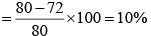QUESTION: 13

The monthly average salary paid to all the employees of a company was ₹ 5000. The monthly average salary paid to male and female employees was ₹ 5200 and ₹ 4200 respectively. Then the percentage of males employed in the company is:



Solution:

Let the number of employees in the company be 100 and the number of  male employees in the company be x.
So, the number of female employees = 100 – x.
According to the question,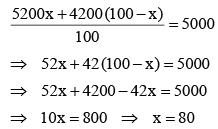So, there are 80% male employees in the company.

QUESTION: 14

In a class, there are 18 very tall boys. If these constitute three-fourths of the boys and the total number of boys is two-thirds of the total number of students in the class, what is the number of girls in the class?



Solution:

Here,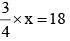x = 24
Boys = 24  and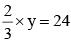y = 36
Total students = 36
The number of girls in the class = 36 – 24 = 12.

QUESTION: 15

Anita’s mathematics test had 70 problems carrying equal marks i.e., 10 arithmetic, 30 algebra and 30 geometry. Although she answered 70% of the arithmetic, 40% of the algebra and 60% of the geometry problems correctly, she did not pass the test because she got less than 60% marks. The number of more questions she would have to answer correctly to earn a 60% passing marks is:



Solution:

Questions correctly answered by Anita are as follows:
Arithmetic – 70% of 10 = 7
Algebra – 40% of 30 = 12
Geometry – 60% of 30 = 18
∴ Total questions correctly answered = 7 + 12 + 18 = 37
Anita had to attempt 60% of 70 questions i.e., 42 questions correctly to pass the test.
∴ Number of more questions required to be answered = 42 – 37 = 5

QUESTION: 16

In aid of charity, every student in a class contributes as many rupees as the number of students in that class. With the additional contribution of ₹ 2 by one student only, the total collection is ₹ 443. Then how many students are there in the class?



Solution:

Let the number of students in the class be x.
Total collection without considering the additional contribution by one student = 443 – 2 = ₹ 441
So,  x × x = 441
⇒ x2 = 441
⇒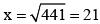QUESTION: 17

30g of sugar was mixed in 180 ml water in a vessel A, 40 g of sugar Was mixed in 280 ml of water in vessel B and 20 g of sugar was mixed in 100 ml of water in vessel C. The solution in vessel B is :



Solution:

Vessel A : 30g sugar mixed in 180ml water.
So, the concentration of sugar in vessel A is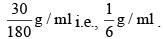Vessel B : 40g sugar mixed in 280ml water.
So, the concentration of sugar in vessel B is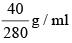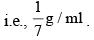Vessel C : 20g sugar mixed in 100ml water.
So, the concentration of sugar in vessel C is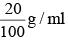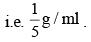More the concentration of sugar, more will be the sweetness.
Therefore, in terms of sweetness : C > A > B

QUESTION: 18

A class starts at 11:00 am and lasts till 2:27 pm. Four periods of equal duration are held during this interval. After every period, a rest of 5 minutes is given to the students. The exact duration of each period is:



Solution:

Class start at 11:00 am
Class ends at 02:27 pm
So, duration of class = 3h 27min = 207 min
There will be 4 periods.
After every period, there will be a 5 min break. Here, only 3 breaks will be taken as there are only 4 periods and end of 4th period means end of the class. 4th break is not needed to be considered.
So, duration of the periods = 207 – 3×5 = 192 min.
∴ Time assigned to each period = 192/4 = 48 min

QUESTION: 19

A person allows 10% discount for cash payment from the marked price of a toy and still he makes a 10% gain. What is the cost price of the toy which is marked ₹ 770?



Solution:

Marked price (M.P.) of toy =  ₹ 770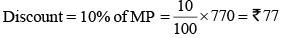Price after discount = ₹ (770 – 77) = ₹ 693
Let cost price (C.P.) of toy be ₹ x.
According to Question,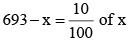⇒ 693 – x = 0.1 x
⇒ 1.1 x = 693 ⇒ x = 630
∴ Cost price of the toy = ₹ 630
MP = 770
SP after discount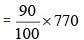CP getting 10% gain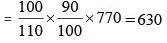QUESTION: 20

A round archery target of diameter 1 m is marked with four scoring regions from the centre outwards as red, blue, yellow and white. The radius of the red band is 0.20 m. The width of all the remaining bands is equal. If archers throw arrows towards the target, what is the probability, that the arrows fall in the red region of the archery target?



Solution: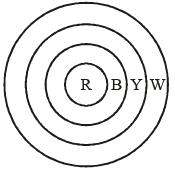We know that, area of a circle = πr2
So, Red band area = π(0.2)2 = 0.04π
All the other than red are in the form of a ring.
So, Blue band area = π((0.3)2 – (0.2)2)
= π((0.3 – 0.2) (0.3 + 0.2)) = 0.05 π Similarly, yellow band area = 0.07 π And white band area = 0.09 π
∴ Required probability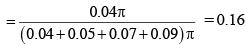Alternate Method:
Area of red circle = π(20)2
Radius of Archry board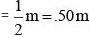Area of Archry Board = π(.50)2